Courses

# NCERT Solutions - Force and Laws of Motion Class 9 Notes | EduRev

## UPSC : NCERT Solutions - Force and Laws of Motion Class 9 Notes | EduRev

The document NCERT Solutions - Force and Laws of Motion Class 9 Notes | EduRev is a part of the UPSC Course NCERT Textbooks (Class 6 to Class 12).
All you need of UPSC at this link: UPSC

Page No. 118

Q.1. Which of the following has more inertia?
(a) A rubber ball and a stone of the same size?
(b) A bicycle and a train?
(c) A five rupees coin and one-rupee coin?
Ans.
(a) Out of a rubber ball and a stone of the same size, the mass of stone is larger; so stone has more inertia than a rubber ball.
(b) Out of a bicycle and a train, train has much larger mass than bicycle, so train has more inertia.
(c) Out of five rupees and one rupee coins, live rupee coin has larger mass and hence more inertia.

Q.2. In the following example, try to identify the number of times the velocity of the ball changes.
"A football player kicks a football to another player of his team who kicks the football towards the goal. The goalkeeper of the opposite team collects the football and kicks it towards a player of his own team": Also identify the agent supplying the force in each case.
Ans.
The velocity of the ball changes four times in the following ways:
(a) Player 1 changes the velocity by kicking it.
(b) Player 2 changes the velocity by kicking it to the goal.
(c) Goalkeeper changes the velocity by, collecting the ball.
(d) Goalkeeper changes the velocity by kicking the ball.

Q.3. Explain why some of the leaves may get detached from a tree if we vigorously shake its branch.
Ans. The leaves of the tree have inertia of rest. When the branch is shaken, it moves, but the leaves tend to be in a state of rest due to their inertia. Thus, they get detached from the tree.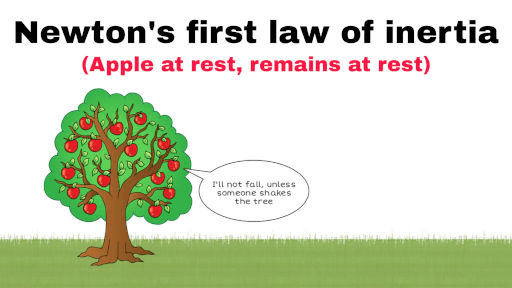Q.4. Why do you fall in the forward direction when a moving bus apply brakes to stop and fall back when it accelerates from rest?
Ans.

• In a running bus, our speed is equal to the speed of the bus. As a moving bus brakes to a stop, the lower part of our body being in contact with the bus comes to rest, but the upper part due to inertia of motion remains in the state of motion.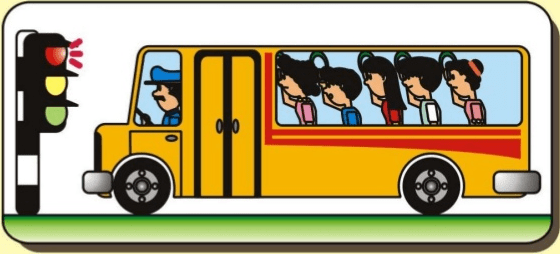The bus stops suddenly, Passenger jerks forward
• Hence, we fall in the forward direction. When the bus accelerates from rest, the feet come into motion while the upper part of the body remains at rest due to inertia of rest; hence, we fall backwards.
Page No. 126

Q.1. If action is always equal to the reaction, explain how a horse can pull a cart.
Ans.

• When a horse pulls a cart, the force exerted by horse on the cart is always equal and opposite to the force exerted by cart on the horse.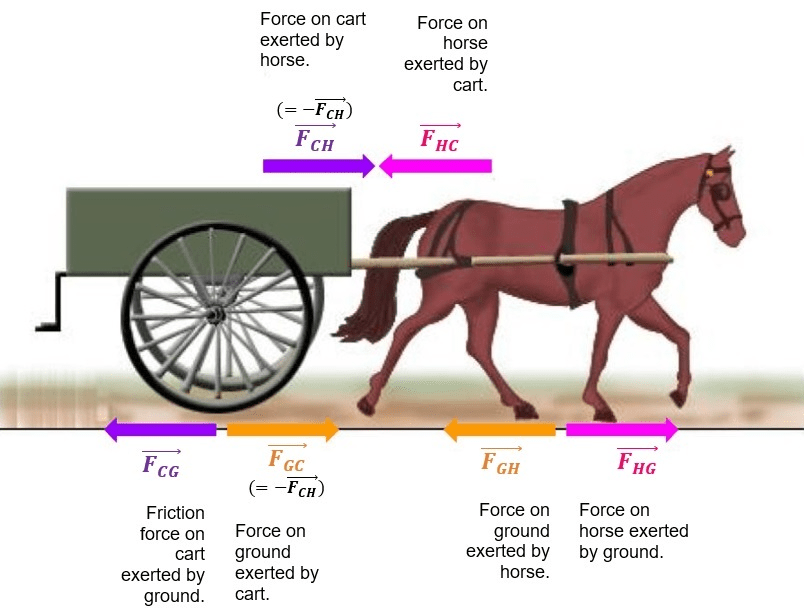• So, at first glance, it seems that the forces being equal and opposite cancel each other out and hence, the cart would not move. It is a fact that the cart moves. Then the question arises why does the cart move? This is possible only when the horse is able to apply enough force to overcome the frictional forces.
• Thus, to make the cart move, the horse bends forward and pushes the ground backward with its feet. When the forward force to the backward push of the horse is greater than the opposing frictional forces between tyres of cart and ground, the cart moves.

Q.2. Explain, why is it difficult for a fireman to hold a hosepipe, which ejects large amounts of water at high velocity.
Ans.

• When a fireman directs a powerful stream of water on fire from a hosepipe; the water stream has huge forward momentum.
• The fireman holding the hose pipe has to balance this momentum by applying a sufficient force in backward direction.
• Therefore, it is difficult for a fireman to hold a hose, which ejects large amount of water at a high velocity.

Q.3. From a rifle of mass 4 kg, a bullet of mass 50 g is fired with an initial velocity of 35 ms-1. Calculate the initial recoil velocity of the rifle.
Ans.
► Mass of rifle, M = 4 kg;

► Mass of bullet, m = 50g = 0.05 kg
► Initial velocity of rifle (U) and bullet (u) = 0.
► Final velocity of bullet, v = 35ms-1
► Recoil velocity of rifle, V = ?
By law of conservation of momentum:
mu + MU = mv + MV
Since u = U = 0,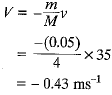Recoil velocity of gun in backward direction = 0.43 m s-1.

Q.4. Two objects of masses 100 g and 200 g are moving along the same line and direction, with velocities 2 ms-1 and 1 ms-1, respectively. They collide and after the collision, the second object moves with a velocity of 1.67 ms-1. Determine the velocity of the first object.
Ans.
► Object 1, Object 2
► Mass, m1 = 100g = 0.1 kg
► m2 = 200g = 0.2 kg
► Initial velocity, u1 = 2 ms-1, u2 = 1 ms-1
► Final velocity, v1 = 1.67 ms-1, v2 = ?
By law of conservation of momentum: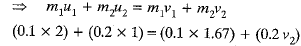⇒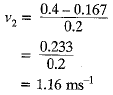Velocity of second object =1.16ms-1

Page No. 128

Q.1. An object experiences a net zero external unbalanced force. Is it possible for the object to be travelling with a non-zero velocity? If yes, state the conditions that must be placed on the magnitude and direction of the velocity. If no, provide a reason.
Ans.

• Yes, it is possible for the object to be travelling with a non-zero velocity, if it experiences a net zero external unbalanced force.
• This is due to inertia of motion. If body is initially moving with some velocity on a smooth surface, then it will continue to move with same velocity, though the net external force acting on the body is zero.
Example: When we stop pedalling a moving bicycle, the bicycle begins to slow down and finally comes to rest. This is again because of the friction forces acting opposite to the direction of motion. The force of friction opposes the motion of the bicycle. If there were no unbalanced force of friction and no air resistance, a moving bicycle would go on moving forever.

Q.2. When a carpet is beaten with a stick, dust comes out of it. Explain.
Ans. When the carpet is beaten, it is suddenly set into motion. The dust particles tend to remain at rest due to the inertia of rest, therefore, dust comes out of it.

Q.3. Why it is advised to tie any luggage kept on the roof of a bus with a rope?
Ans. The luggage kept on the roof possesses inertia. As the bus moves, breaks to stop or takes a turn, the luggage might fall off. Thus, it should be tied with a rope.

Q.4. A batsman hits a cricket ball which then rolls on level ground. After covering a short distance, the ball comes to rest. The ball slows to a stop because
(a) the batsman did not hit the ball hard enough.
(b) velocity is proportional to the force exerted on the ball.
(c) there is a force on the ball opposing the motion.
(d) there is no unbalanced force on the ball, so the ball would want to come to rest.
Ans. (c) there is a force on the ball opposing the motion.

Q.5. A truck starts from rest and rolls down a hill with a constant acceleration. It travels a distance of 400 m in 20 s. Find its acceleration. Find the force acting on it if its mass is 7 metric tonnes
(Hint : 1 metric tonne = 1000 kg.)
Ans.
Initial velocity of truck (u) = 0
Time (t) = 20 s
Distance covered (S) = 400 m
Acceleration (a) = ?
Mass of truck (m) 7 tonne = 7000 kg
Force on truck We know ; (F) = ?
We know:
► S = ut  1/2 at2
► 400 = 0 × 20 1/2 × a × (20)2
► 400 = 200a
► a = 2ms-2
Force on truck (F) = ma
= 7000 x 2
= 14000 N

Q.6. A stone of 1 kg is thrown with a velocity of 20 ms-1 across the frozen surface of a lake and comes to rest after travelling a distance of 50 m. What is the force of friction between the stone and the ice?
Ans.
Mass, m = 1kg; Initial velocity, u = 20 ms-1
► Final velocity, v = 0;
► distance travelled, s = 50 m
► Acceleration,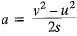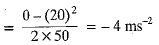► Force exerted = ma
= 1 kg x (- 4) ms-2 = - 4N
► Friction = 4 N against the direction of motion.

Q.7. An 8000 kg engine pulls a train of 5 wagons, each of 2000 kg, along a horizontal track. If the engine exerts a force of 40000 N and the track offers a frictional force of 5000 N, then calculate:
(a) the net accelerating force
(b) the acceleration of the train, and
(c) the force of wagon 1 on wagon 2.
Ans.
(a) Force exerted by the engine = 40,000 N
► Force of friction exerted by the tracks = 5000 N
As the force of friction always acts opposite to the direction of applied force.
Net accelerating force of engine:
= 40000 - 5000 = 35000 N
(b)Let us consider the acceleration of the train be a m/s2

Acceleration is the ratio of net acceleration force by mass, i.e

►a = Fa/m

Where m is the mass of the train, it can be calculated as follows

Total mass of the train (m) = Mass of the engine + (Mass of the wagons  × Number of wagons)

m = 8000 + (5 × 2000)

m = 18000 kg

Acceleration of the train (a) = 35000/18000

a = 1.944m/s2

(c) Force of wagon 1 on wagon 2
► F = ma
= 8000 × 3.5 = 28000 N

Q.8. An automobile vehicle has a mass of 1500 kg. What must be the force between the vehicle and road if the vehicle is to be stopped with a negative acceleration of 1.7 ms-2?
Ans. Here, mass of automobile, m = 1,500 kg
Acceleration, a = 1.7 ms-2 force between the vehicle and road
► F = ma = 1500 kg x ( -1.7 ms-2)
= -2,550 kg ms-2
= -2,550 N

(Negative sign indicates that the force is in a direction opposite to the motion of the vehicle.)

Q.9. What is the momentum of an object of mass m, moving with a velocity v?
(a) (mv)2 (b) mv2 (c) 1/2mv2 (d) mv
Ans. (d) mass x velocity

Q.10. Using a horizontal force of 200 N, we intend to move a wooden cabinet across a floor at a constant velocity. What is the friction force that will be exerted on the cabinet?
Ans.  Frictional force should balance with the applied horizontal force.
Thus friction = - 200 N

Q.11. Two objects, each of mass 1.5 kg, are moving in the same straight line but in opposite directions. The velocity of each object is 2.5 ms-1 before the collision during which they stick together. What will be the velocity of the combined object after the collision?
Ans. Mass of each object m1 = m2 = 1.5 kg.
Initial velocity of first object, u1 = 2.5 ms-1
Initial velocity of second object, u2 = - 2.5 ms-1
Combined mass after collision = m1 + m2 = 1.5 + 1.5 = 3.0 kg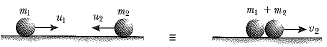From the principle of conservation of momentum: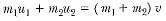⇒ 1.5 x 2.5 + 1.5 x (-2.5) = (3.0) v
⇒ 0 = 3.0 v
or v = 0 ms-1
That is combined object comes to rest after collision.

Q.12. According to the third law of motion when we push on an object, the object pushes back on us with an equal and opposite force. If the object is a massive truck parked along the roadside, it will probably not move. A student justifies this by answering that the two opposite and equal force cancel each other Comment on this logic and explain why the truck does not move.
Ans. The logic given by student is wrong; because the action and reaction forces do not act on same body; but act on different bodies.
The reason for not moving the truck is as follows:

• The truck is massive, so it has very large inertia. Moreover, it is parked on the ground; there is a frictional force between the truck and ground.
• The force exerted by us on the truck is insufficient to overcome the force of friction; so these are balanced forces on the truck; (force exerted by us plus force of friction in opposite direction); that is net force is zero; and hence, the truck does not move.

Q.13. A hockey ball of mass 200 g travelling at 10 ms-1 is struck by a hockey stick so as to return it along its original path with a velocity at 5 ms-1. Calculate the change of momentum occurred in the motion of the hockey ball by the force applied by the hockey stick.
Ans. Mass of ball (m) = 200 g = 0.2 kg
► Initial velocity of ball (u1) = 10 ms-1
► Final velocity of ball (u2) = 5 ms-1
(Negative sign denotes that ball is moving in opposite direction)
► Initial momentum of ball = mu1 = 0.2 × 10 = 2 Ns
► Final momentum of ball = mu2 = 0.2 × (- 5) = -1 Ns

Change in momentum = Final momentum + Initial momentum

= 1 + 2 = 3 Ns

Negative sign denotes that change in momentum is in the direction opposite to the direction of initial momentum of the ball.

Q.14. A bullet of mass 10g travelling horizontally with a velocity of 150 ms-1 strikes a stationary wooden block and comes to rest in 0.03s. Calculate the distance of penetration of the bullet into the block. Also, calculate the magnitude of the force exerted by the wooden block on the bullet.
Ans.
Mass of bullet (m) = 10 g = 0.01 kg
Initial velocity of bullet (u) = 150 ms-1
Final velocity of bullet (v) = 0
Time (t) = 0.03 secs
Acceleration on bullet (a) = ?
Force acting on wooden block (F) = ?
Distance penetrated by bullet (s) = ?
We know:
v = u + at
► 0 = 150 + (a × 0.3)
► a × 0.03 = 150
► a = 5000ms-2
We know:

S = ut + 0.5at2
= 150 × 0.03 + (5000) × (0.03)2 = 4.5 + 2.25 = 6.75m
We know:
F = ma
Force acting on bullet (F) = 0.01 × (5000) = 50N
Negative sign denotes that wooden block exerts force in the direction, opposite to the direction of motion of the bullet.

Q.15. An object of mass 1 kg travelling in a straight line with a velocity of 10 ms-1 collides with and sticks to a stationary wooden block of mass 5 kg. Then they both move off together in the same straight line. Calculate the total momentum just before the impact and just after the impact. Also, calculate the velocity of the combined object.
Ans.
For object:
m1 = 1 kg
u= 10 ms1
For wooden block:
m2 = 5 kg
u2 = 0
Momentum just before collision:
= m1u1 + m2u2
= 1 × 10 + 5 × 0
= 10 kg ms-1
Mass after collision = (m+ m2)
= 1 + 5 = 6 kg
Let velocity after collision = v
Momentum after collision = 6 × v
Using the law of conservation of momentum:
Momentum after collision = Momentum before collision
► 6 × v = 10
► v = 1.67 ms-1

Q.16. An object of mass 100 kg is accelerated uniformly from a velocity of 5 ms-1 to 8 ms-1 in 6s. Calculate the initial and final momentum of the object. Also, find the magnitude of the force exerted on the object.
Ans.
► Mass of object (m) = 100 kg
► Initial velocity (u) = 5 ms-1
► Final velocity (v) = 8 ms-1
Time (t) = 6 s
► Initial momentum (Pinitial) = mu = 100 × 5 = 500 Ns
► Final momentum (Pfinal) = mv = 100 × 8 = 800 Ns
► Force exerted on the object (F) = [(mv - mu) / t] = (800 - 500)/6 = 300/6 = 50N

Q.17. Akhtar, Kiran and Rahul were riding in a motorcar that was moving with a high velocity on an expressway when an insect hit the windshield and got stuck on the windscreen. Akhtar and Kiran started pondering over the situation. Kiran suggested that the insect suffered a greater change in momentum as compared to the change in momentum of the motorcar (because the change in the velocity of the insect was much more than that of the motorcar). Akhtar said that since the motorcar was moving with a larger velocity, it exerted a larger force on the insect. And as a result the insect died. Rahul while putting an entirely new explanation said that both the motorcar and the insect experienced the same force and a change in their momentum. Comment on these suggestions.
Ans. Explanation given by Rahul is correct. Both insect and motor car exert same force on each other as action and reaction forces are equal. Thus, their momenta also experience equal change in opposite direction.

Q.18. How much momentum will a dumb-bell of mass 10 kg transfer to the floor if it falls from a height of 80 cm and does not rebound? Take its downward acceleration to be 10ms-2.
Ans.
Height, h = 80 cm = 0.8 m
Mass, m = 10 kg ; Initial velocity, u = 0
► Acceleration, a = 10 ms-2
► Final velocity, v =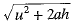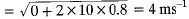► Momentum transferred = mv = 10 kg x 4 ms-1
= 10 kg x 4 ms-1
= 40 kgms-1

Page No. 130

Q.1. The following is the distance-time table of an object in motion time in seconds Distance in meters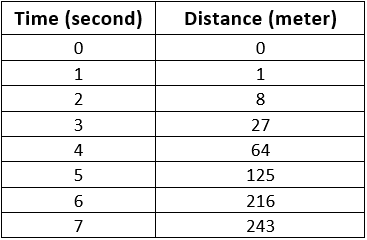(a) What conclusion can you draw about the acceleration? Is it constant, increasing, decreasing or zero?
(b) What do you infer about the forces acting on the object?
Ans.
(a) Initial speed, u = 0
Since s =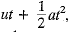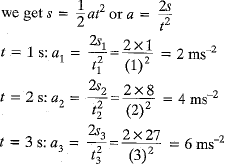Thus, acceleration is increasing with time.
(b) Force, F = ma ⇒ F ∝ a
Since acceleration is increasing with time, force is also increasing with time.

Q.2. Two persons manage to push a motorcar of mass 1200 kg at a uniform velocity along a level road. The same motorcar can be pushed by three persons to produce an acceleration of 0.2 ms-2. With what force does each person push the motorcar? (Assume that all persons push the motorcar with the same muscular effort.)
Ans.
Mass of car, m = 1200 kg
Let each person applies force F on the motor car. Thus, the force ‘2F applied by 2 persons balances the frictional fore due to ground force applied by three persons = 3F
Effective force = 3F - friction = 3F - 2F = F
Thus force F produces an acceleration of 0.2 ms-2.
F = ma = 1200 x 0.2 = 240 N
Thus, force applied by each person = 240 N

Q.3. A hammer of mass 500 g, moving at 50 ms-1, strikes a nail. The nail stops the hammer in a very short time of 0.01 s. What is the force of the nail on the hammer?
Ans. Mass of hammer, M = 500 g = 0.5 kg
Initial velocity of hammer, u = 50 ms-1
Final velocity of hammer, v = 0
Time, t = 0.01 s
Acceleration,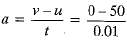= - 5000 ms-2
Force applied by nail, F = ma
= (0.5) x (-5000 ms-2)
= - 2500 N (opposite to motion of hammer)

Q.4. A motorcar of mass 1200 kg is moving along a straight line with a uniform velocity of 90 km/h. Its velocity is slowed down to 18 kmh-1 in 4s by an unbalanced external force. Calculate the acceleration and change in momentum. Also, calculate the magnitude of the force required.
Ans.
► Mass of motor car, m = 1200 kg
► Initial velocity of car, u = 90 km/h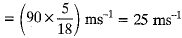► Final velocity of car, v = 18 km/h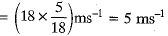Time, t = 4s
► Acceleration,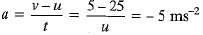Momentum change = mv - mu
= 1200 (5 - 25) = - 24000 kg ms-1
Magnitude of force, F = ma
= 1200 x (- 5) = - 6000 N
Acceleration, momentum change and force are opposing the motion of motorcar, as indicated by negative sign.

Offer running on EduRev: Apply code STAYHOME200 to get INR 200 off on our premium plan EduRev Infinity!

## NCERT Textbooks (Class 6 to Class 12)

954 docs|585 tests

,

,

,

,

,

,

,

,

,

,

,

,

,

,

,

,

,

,

,

,

,

;